Electrochemical (2018 NHT) 1) Motor vehicles, such as cars, are a standard means of transport around the world. The majority of cars use a battery to start the engine and then use the combustion of a fuel in the engine to power the car. The standard battery used to start a car is a rechargeable lead-acid battery. The components of a simplified lead-acid battery are shown below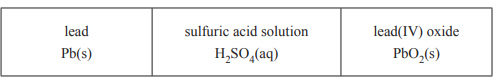When the battery is fully charged, it has a lead, Pb, anode, a lead(IV) oxide, PbO2, cathode and a sulfuric acid, H2SO4, electrolyte. When fully discharged, the two electrodes are both coated in lead(II) sulfate, PbSO4 .a) Write the oxidation reaction, including states, that occurs during discharge. b) Write the reduction reaction, including states, that occurs during discharge. Solutionc) The car is powered by the combustion of a fuel in the engine and, historically, the fuel has been petrol. Ethanol, C2H5OH, and hydrogen, H2, are now being used as alternative fuels in cars. A significant component of petrol is 2,2,4-trimethylpentane, C8H18, which has the same molar heat of combustion as octane, C8H18. i. Write the balanced thermochemical equation for the combustion of C8H18 in excess oxygen, O2. ii. Calculate the mass of carbon dioxide, CO2., in kilograms, released during the complete combustion of 1.00 kg of C8H18 M(C8H18) = 114 g mol–1 Solutioniii. Calculate the mass of CO2, in kilograms, released during the combustion of 1.00 kg of C2H5OH. M( C2H5OH) = 46 g mol–1 Solution iv. Both petrol and ethanol produce about 15.50 MJ of energy for each kilogram of CO2 produced. Use any information in parts a, b or c above to describe one advantage of using petrol as a fuel. Solutiond) An alternative means of providing power to cars is the combustion of H2 in fuel cells. i. If 582 L of H2 (measured at 100 kPa and 18 °C) were combusted, what amount of energy, in megajoules, would be released? Solution   ii. H2 can be produced by several methods. Two of these methods are given below. Method 1 Reacting methane with water at a high temperature CH4(g) + 2H2O(g) --> CO2(g) + 4H2(g) Method 2 The electrolysis of water 2H2O(l) --> O2(g) + 2H2(g) Evaluate the environmental impacts of each of these methods of producing H2. Solution2) A zinc-carbon dry cell battery has a potential of +1.50 V measured at standard conditions. The two half-reactions that occur in this battery are shown in the following equations.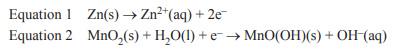Assuming standard conditions, the electrode potential of Equation 2 is A. +2.26 V B. +0.74 V C. –0.74 V D. –2.26 V Solution3) Which one of the following produces heat energy as its main energy output?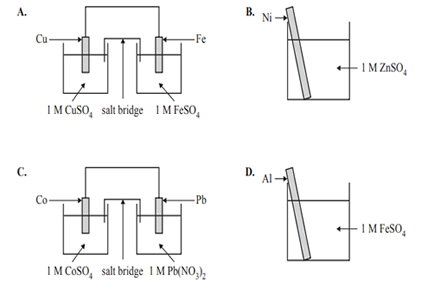Solution4) KOH is used as part of a rechargeable nickel-cadmium, NiCd, battery. The chemical reactions that occur in an NiCd battery during discharge are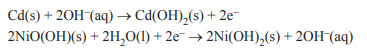i. Identify the reducing agent in these reactions during discharge. ii. Identify the oxidising agent in these reactions during recharge. iii. What is the purpose of the KOH in the NiCd battery? Solution5) The following diagrams represent the operation of a secondary cell during recharge and discharge, in no particular order. The diagrams of the circuits are not complete.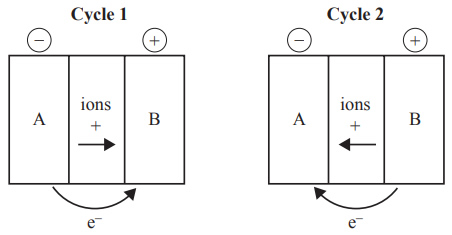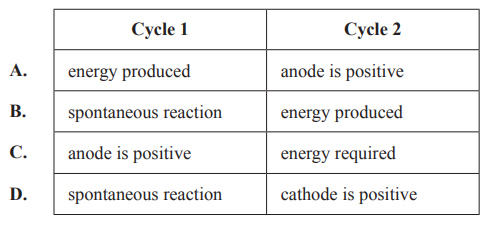Solution